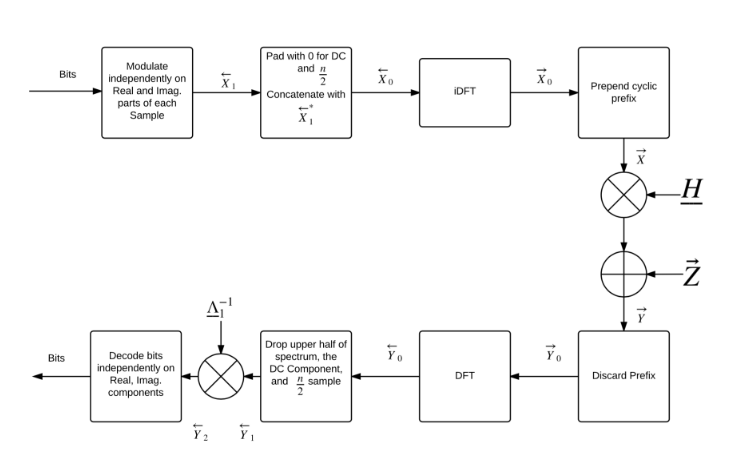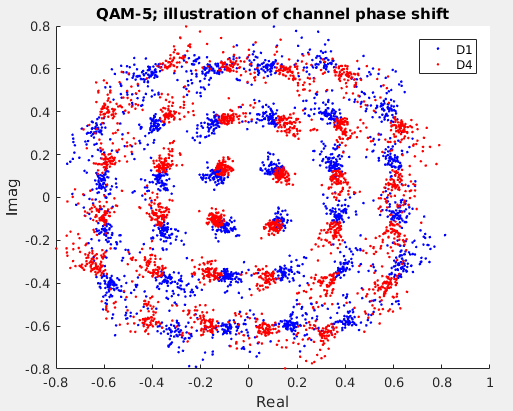## Friday, June 16, 2017

### A digital communication project using OFDM and 32-QAM

This past semester (my last one during my undergrad years!), I was enrolled in ECE4670 -- Intro to Digital Communication with Professor Aaron Wagner. The final project required constructing the encoder and decoder (in Matlab) to send data over an existing channel (Cornell’s commcloud server). The project was done in groups of 2 and I had worked with my friend Balazs Szegletes. It was a fun learning experience, and I wanted to share the details of the project here!

The communication channel is effectively a DAC that outputs the results of the encoder, X, a low-pass filter, and then an ADC that samples this and sends data, Y, to the decoder. The means for sending data to the channel involves writing the data to a wav file, which is then passed as an input parameter to a program called ccplay that is provided for the class. The program handles the DAC and ADC and saves the sampled output as another wav file. The goal is to be able to push data through the channel as quick as possible with few number of bit errors. The figure of merit is computed as:
FOM = (min(R, 300000)*(1-N/50000)10)/max(1,400*Po)
Where R is the data rate defined as (100,000/(L/44100)) where L is the length of the output of the encoder
N is the number of bit errors
Po is the average transmitted power

The channel properties can be modeled by the Toeplitz matrix H, such that:
Y = HX + Z, where Z is iid (independent, identically distributed) Gaussian noise. The commcloud server has two such channels, audio0 and audio1.

This builds on a previous lab, where orthogonal frequency division multiplexing (OFDM) is used with on/off keying to send data over the channel. This scheme achieved a data rate of about 14,000 bits per second with zero errors, resulting in a figure of merit of about 14,000. The high performance design utilizes orthogonal frequency division multiplexing (OFDM) and quadrature amplitude modulation (QAM) to achieve a figure of merit much higher than the previous lab.

The overall OFDM system block diagram is shown below (taken from Professor Wagner’s course’s Scribe notes):The big gain in performance stems from the use of QAM, which allows encoding multiple bits per sample, in the complex plane. A modulation order (M) of 5 was selected for the design, ie 32-QAM. This was experimentally determined to be the optimal modulation order, which gives the highest data rate (R) before bit errors (N) start outweighing the benefits from a higher data rate.

The encoder encodes the incoming bit-stream into frequency components and then processes it as described below before sending out its time-domain signal over the channel.

The encoder accepts the input vector (of length 100,000) and encodes the vector using 32-QAM into 100,000/5 complex samples in a vector x. The encoder then separates x into 8 equal sized data blocks D1...D8 of length L1. The size of L1 is determined by modulation order M: L1=(100,000/(8*5)). Two training blocks, T1 and T2, each a vector consisting all 1’s and of length L1,  are inserted to create the following vector, x, of length 10L1.

Using a seed of 1, and Matlab’s rand function, a vector, p1, of pseudorandom phase angles (between 0 and 2 pi) is generated. These are used to create a vector p2 of phasors, where:
p2(i) = gamma*exp(1jp1(i)) for all i = indices of elements of p1,p2. The constant gamma sets the average power level.

The elements of this vector are then multiplied element-wise with the elements in vector x. This is to prevent the constructive addition of several frequency components in the time domain which would cause the time-domain signal to clip or saturate.

Each block is then prepended by NZpre zero’s. NZpost zero’s are appended to each block. Let this vector be called X. X is then padded with 0 for DC and concatenated with X*. This ensures that the time domain signal is real-valued. The inverse DFT of this is taken to get the real-valued time domain signal. Each block is prepended with a cyclic prefix (length Kc) to make the (Toeplitz) channel transformation effectively look circulant. The encoder then outputs this vector.

The training blocks are inserted into the data vector so that it may be used at the decoder end for training of channel properties. Each element of a training block (in the frequency domain) has magnitude 1 and a phase theta_t. When this element goes through the channel, it is attenuated and incurs a phase shift and is presented to the decoder with magnitude gamma_c(attenuation of the channel) and phase (theat_t+theta_c) where theta_c is the phase added by the channel. Each frequency component has an associated attenuation and phase addition. Since the training block is the same length as any individual data block, there exists known training information about attenuation and phase for each frequency of interest in the system/encoding at the decoder end. The decoder is then able to use this block’s received data to cancel channel effects for the other blocks. However, it must be noted that the the attenuation and phase addition of the channel are not static and vary with time. Thus it is important not to let the training data be stale. This is the reason two training blocks are used, and also why they are inserted in the order they are, with respect to the data blocks.

It was observed that placing only one training block at the beginning of the data vector allows determining channel phase reasonably well for only a couple data blocks adjacent to it. Additionally, it was noted that decreasing the number of blocks also made the prediction worse. By placing the two training blocks as they are in the vector allows using a block to cancel channel effects for two adjacent data blocks. The phase variation from the training data to the two data blocks is small enough to allow sufficiently high figure of merit. The phase variation from D1 to D4 is illustrated below:While the shift is somewhat significant, it is still possible to correctly decode the information. As can be imagined, too far from this and the bit error rate gets terrible.

At the decoder end, synchronization is performed to determine the start of the signal. This is done by thresholding the average power level over five samples. To recover the samples encoded in the frequency domain, the decoder reverses what the encoder did, firstly ignoring the cyclic prefix, performing a DFT, dropping the flipped conjugate second half of the vector and then dropping the zero-padding. Since gamma_c*exp(1j*theta_c) is known from the training block for each of the frequency levels, the samples in D1 to D4 are divided by gamma_c*exp(1j*theta_c) from T1 to cancel the channel effects. Similarly, D5 to D8 use the channel information from T2. Finally, the pseudo-random phases added at the encoder side are removed by dividing by each of the known phases element-wise. These phases are known since the random number generator had a fixed seed of 1. The individual data blocks are then demodulated (QAM) and concatenated to each other to form the 100,000 long bit vector. For modulating and demodulating using QAM, Matlab’s inbuilt functions qammod and qamdemod are used.

The following constants were determined to give the highest figure of merit:
NZpre = 110
NZpost = 1050
gamma =7.8 (chosen to meet the power constraint of 1/400)
Kc = 170

Experimentally, the figure of merit scores are about 44,000, and 56,000 for channels audio0 and audio1 respectively. The constants NZpre, NZpost, gamma, modulation order M, number of data blocks, number of training blocks, and placement of training block were all experimented with and the results presented here represent the best combination for the highest figure of merit. With only one training block, the phase drift was high enough to negate the benefits of higher data rate obtained from 32-QAM. However, adding a third training block was seen as a waste since it would minimally reduce the number of bit errors but would hurt the data rate appreciably. Using convolutional coding was considered but it was determined as not worth the effort given the performance obtained and the added complexity.

I expect to write another post soon to discuss some technical background behind OFDM, training and synchronization. Let me know what you think and if there's something else from the project you'd like me to cover in more detail!